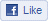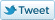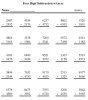This browser does not support IFRAMEs - no Ads will appearBlueBonkers Home  >  Math Sheets Home  >   Fractions Practice SheetsBB on FacebookBB on Twitter

# Fractions Practice Sheets

( printable math practice pages)

Our Fractions practice sheets are custom made and feature various degrees of difficulty ! Each practice fractions math sheet also has an associated answer sheet.

We have organized our practice math sheets so that you can work your way through them and always keep track of where you left-off (notice the numbering system). This allows you to always have access to fresh content with no fear of duplication! Browse around, and then try a few of our FREE printable fractions practice sheets at home (or in your classroom)!Practice math sheets in this category include:

• Add Simple Fractions with like denominators
• Add Simple Fractions with unlike denominators
• Add Mixed Fractions with like denominators
• Add Mixed Fractions with unlike denominators
•Subtraction of Fractions

Practice math sheets in this category include:

• Subtract Simple Fractions with like denominators
• Subtract Simple Fractions with unlike denominators
• Subtract Mixed Fractions with like denominators
• Subtract Mixed Fractions with unlike denominators
•Multiplication of Fractions

Practice math sheets in this category include:

• Multiplication Simple Fractions with like denominators
• Multiplication Simple Fractions with unlike denominators
• Multiplication Mixed Fractions with like denominators
• Multiplication Mixed Fractions with unlike denominators
•Division of Fractions

Practice math sheets in this category include:

• Division Simple Fractions with like denominators
• Division Simple Fractions with unlike denominators
• Division Mixed Fractions with like denominators
• Division Mixed Fractions with unlike denominators
•Reduction of Fractions

Practice math sheets in this category include:

• Reduce Fractions with single-digit numerators
• Reduce Fractions with 2-digit numerators
• Improper/Mixed Fractions with no preceeding integer
• Improper/Mixed Fractions with preceeding integer
•

All of our pages are easily printable (without advertisements) from your "local" printer (one attached to your computer). Most pages have a button or link that is marked "Print" or "Print this Page". All pages are configured for best viewing and print capabilities using the MS-IE (Microsoft, Internet Explorer) browser ver 6.0 or higher.